# Symmetric Kruskal Tensors

A symmetric Kruskal tensor is a decomposition of a tensor into a sum of vector outer products. The symmetric structure means that each term in the summand is the outer product of a single vector with itself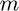times, whereis the number of modes of the decomposed tensor. This contrasts with a generic Kruskal tensor, where each summand is an outer product of m different vectors. More concisely, a symmetric Kruskal tensor decomposition of a tensor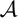has the following form: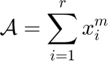In this notation, a subscript refers to a column index. A superscript refers to the outer product of a single vector with itselftimes.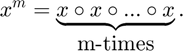The number of summands in the decomposition,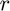, is referred to as the number of components of the symmetric Kruskal tensor.

An alternative, often equivalent expression for a symmetric Kruskal tensor decomposition specifies a real-valued weight for each of the summands in the outer product. The-vector formed by these weights is referred to as the weight or lambda vector of the symmetric Kruskal decomposition.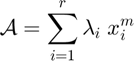In certain cases the lambda vector is required in order for a symmetric Kruskal decomposition to exist, e.g. when a symmetric Kruskal tensor has an even number of components and the tensor to be decomposed has a negative element on its main diagonal. In many other cases, the lambda vector is optional and the symmetric Kruskal decomposition can be represented without specifying a lambda vector.

The symktensor class stores symmetric Kruskal tensors, and exploits the extra symmetric structure to perform many calculations more efficiently.

## Declaring a symmetric Kruskal tensor with symktensor

The symktensor format stores the vectors and weights of a symmetric Kruskal tensor decomposition. The vectors in the decomposition are collected as the columns of a matrix X, referred to as the factor matrix. The lambda vector, containing the (often optional) weights is input into the constructor as a column vector. The lambda vector and factor matrix are collectively referred to as the constituent parts in the declaration of a symktensor. For example, consider the decomposition of a tensor.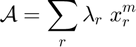In the example that follows, we form a symmetric Kruskal decomposition by specifying a factor matrix, lambda vector, and the number of modes of the decomposed tensor. We pass all three arguments to the symktensor constructor. This can be stored as a symmetric Kruskal tensor as follows.

n = 4; %The dimension in each mode of the tensor A
m = 3; %The number of modes of A
r = 2; %The rank of the decomposition
X = reshape(1:n*r,n,r); %The columns of this matrix are the vectors in decomposition
L = [1; -1]; %the weights (should be a column vector of length r)
S = symktensor(L, X, m) %Declare a symktensor object

S is a symktensor of order 3 and dimension 4
S.lambda = [ 1 -1 ]
S.U =
1     5
2     6
3     7
4     8


A symktensor object can be declared without a weight vector by specifiying the number of modes, the rank, and an additional 'nolambda' option. In this case, the lambda vector is set to a vector of all ones.

S2 = symktensor(X, m, r, true)

S2 is a symktensor of order 3 and dimension 4
S2.lambda = [ 1  1 ]
S2.U =
1     5
2     6
3     7
4     8


A random symktensor object can be declared by passing the constructor two arguments: the rank of the decomposition and a tensor or symtensor (for size). The lambda vector is taken to be all ones, and the factor matrix has elements drawn uniformly from (0,1).

T1 = tensor(n*ones(1,m)); %<-- Declare a tensor for size
T2 = symtensor(@ones, n,m); %<-- Declare a symtensor for size
rng('default'); %<- Setting random seed for reproducibility of this script

S2 = symktensor(r, T1) %<--Declare a random symktensor from tensor for size
S2 = symktensor(r, T2) %<--Declare a random symktensor from symtensor for size

S2 is a symktensor of order 2 and dimension 1
S2.lambda = [ 1  1 ]
S2.U =
0.8147    0.9058
S2 is a symktensor of order 4 and dimension 3
S2.lambda = [ 1  1 ]
S2.U =
0.1270    0.0975
0.9134    0.2785
0.6324    0.5469


This method of randomly generating a symktensor is useful when setting an initialization point in symmetric decomposition methods (i.e. cp_sym).

Lastly, a symktensor object can be declared from a vectorized version of the factor matrix and lambda vector, in which the lambda vector is stacked on top of a vectorized version of the factor matrix. The shape of the tensor must also be specified, by either passing a tensor/symtensor or listing the number of modes and the rank of the decomposition explicitly. Additionally, a 'nolambda' option can be added to any of these constructions, in which case the lambda vector should not be stacked onto the factor matrix.

V = [L; X(:)]; %<--Forming the vectorized version
S2 = symktensor(V, symtensor(@ones,m,n)) %<--size specified from symtensor

S2 = symktensor(X(:), symtensor(@ones,m,n), true) %<--'nolambda' option

S2 = symktensor(V, m, r) %<--size specified from modes and dimension

S2 = symktensor(X(:), m, r, true) %<--size from modes and dimension, 'nolambda' option

S2 is a symktensor of order 3 and dimension 4
S2.lambda = [ 1 -1 ]
S2.U =
1     5
2     6
3     7
4     8
S2 is a symktensor of order 3 and dimension 4
S2.lambda = [ 1  1 ]
S2.U =
1     5
2     6
3     7
4     8
S2 is a symktensor of order 3 and dimension 4
S2.lambda = [ 1 -1 ]
S2.U =
1     5
2     6
3     7
4     8
S2 is a symktensor of order 3 and dimension 4
S2.lambda = [ 1  1 ]
S2.U =
1     5
2     6
3     7
4     8


A symmetric Kruskal tensor can also be constructed directly from a generic Kruskal tensor in the ktensor format. If the Kruskal tensor is not symmetric, it is symmetrized by averaging the factor matrices and taking care to get the signs aligned.

K = ktensor(L, X-1, X+2, 2*X);
S2 = symktensor(K)

S2 is a symktensor of order 3 and dimension 4
S2.lambda = [ 1  1 ]
S2.U =
1.2220   -6.5523
2.5685   -7.8311
3.9151   -9.1100
5.2616  -10.3889


This method of declaring a symktensor is useful in comparing decomposition methods: this constructor allows any decomposition method which generates a ktensor CP model to also generate a symktensor. In this way, decomposition methods which are non-symmetric in nature may easily be applied to symmetric problems.

## Use ndims and size for the dimensions of a symktensor

For a given symktensor, ndims returns the number of dimensions (i.e. the number of modes) of the symmetric Kruskal tensor. size returns a size array of the symmetric Kruskal tensor.

%Declaring a symmetric Kruskal tensor
ndims(S)
size(S)

ans =

3

ans =

4     4     4



## Use ncomponents for the rank of symktensor

The function ncomponents returns the number of components of a symktensor object. This isin the symktensor's definition, the number of outer-product summands in the symmetric Kruskal tensor decomposition.

ncomponents(S)

ans =

2



## Use full to convert a symktensor to a tensor

The function full converts a symktensor to a tensor.

full(S)

ans is a symmetric tensor with 3 modes of dimension 4
(1,1,1)  -124
(1,1,2)  -148
(1,1,3)  -172
(1,1,4)  -196
(1,2,2)  -176
(1,2,3)  -204
(1,2,4)  -232
(1,3,3)  -236
(1,3,4)  -268
(1,4,4)  -304
(2,2,2)  -208
(2,2,3)  -240
(2,2,4)  -272
(2,3,3)  -276
(2,3,4)  -312
(2,4,4)  -352
(3,3,3)  -316
(3,3,4)  -356
(3,4,4)  -400
(4,4,4)  -448


## Use double to convert a symktensor to a multi-dimensional array

The function double converts a symktensor to a multi-dimensional array.

double(S)

ans(:,:,1) =

-124  -148  -172  -196
-148  -176  -204  -232
-172  -204  -236  -268
-196  -232  -268  -304

ans(:,:,2) =

-148  -176  -204  -232
-176  -208  -240  -272
-204  -240  -276  -312
-232  -272  -312  -352

ans(:,:,3) =

-172  -204  -236  -268
-204  -240  -276  -312
-236  -276  -316  -356
-268  -312  -356  -400

ans(:,:,4) =

-196  -232  -268  -304
-232  -272  -312  -352
-268  -312  -356  -400
-304  -352  -400  -448



## Basic operations with symktensors

Symktensors support multiplication by scalars. The result is the symktensor with the weight vector multiplied by the scalar.

4*S

ans is a symktensor of order 3 and dimension 4
ans.lambda = [ 4 -4 ]
ans.U =
1     5
2     6
3     7
4     8


## Use norm to compute the Frobenius norm of a symktensor

The function norm returns the Frobenius norm of a symktensor.

norm(S)

ans =

2.1469e+03



## Use normalize to normalize the components of a symktensor.

The function normalize divides each of the columns in a factor matrix by its vector 2-norm. The 2-norm weight is then absorbed into the weight vector of that column.

normalize(S)

ans is a symktensor of order 3 and dimension 4
ans.lambda = [ 164.31677      2295.2176 ]
ans.U =
0.1826   -0.3790
0.3651   -0.4549
0.5477   -0.5307
0.7303   -0.6065


By passing an additional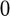argument to the normalize function, the weight vector is set to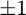and the weights are absorbed into the factor matrix.

normalize(S,0)

ans is a symktensor of order 3 and dimension 4
ans.lambda = [ 1  1 ]
ans.U =
1.0000   -5.0000
2.0000   -6.0000
3.0000   -7.0000
4.0000   -8.0000


## Use arrange to normalize and sort a symktensor

The function arrange normalizes the components of symktensor and sorts them according to the weight vector, in descending order.

arrange(S)
% Additionally, one can pass a permutation array of number of components of
% S. In this case the components are arranged according to the permutation.
arrange(S,[2 1])

ans is a symktensor of order 3 and dimension 4
ans.lambda = [ 2295.2176      164.31677 ]
ans.U =
-0.3790    0.1826
-0.4549    0.3651
-0.5307    0.5477
-0.6065    0.7303
ans is a symktensor of order 3 and dimension 4
ans.lambda = [ -1  1 ]
ans.U =
5     1
6     2
7     3
8     4


## Computing the score of the match between two symktensors

The function score provides a measure of similarity between two symktensors. Given two symktensors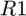and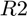, we denote by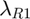and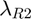their respective weight vectors and X and Y their respective factor matrices. The function score(R1,R2) first normalizes the symtensor. It then attempts to match the symktensortoand returns the following numeric quantification of their similarity.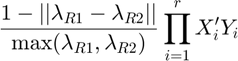In the above formula,is the number of components of.must have at least as many components as. Any additional components are ignored in the score calculation. Since the formula for score depends on the arrangement of the components of, score rearrangesand tries a number of permuations. By default,is rearranged by permuting indices greedily to increase the score. Calling score on two symktensors converts the symktensors to ktensors and calls the |score

R1 = symktensor([1; -1; 1], reshape(1:9, 3, 3), 3); %Declare some symtensors
R2 = symktensor([1; -1], reshape(1:6, 3,2), 3);

score(R1, R2) %The score is 1 (perfect match) because the 1st 2 components of R1 match those of R2

ans =

1



Calling score on two symktensor converts the symktensors to ktensors and calls the score function for ktensor. See the ktensor/score documentation for more information.

## Subscripted reference for symktensors

After defining a symktensor, one can reference its weight vector, factor matrix, or element using the following conventions. Note that elements are queried using multi-dimensional subscript notation, as opposed to linear.

S.lambda %<-- The weight vector
S.X %<-- The factor matrix

S(1,2,1) %<-- Generate the element of index (1,2,1) from the factorization

ans =

1
-1

ans =

1     5
2     6
3     7
4     8

ans =

-148



## Subscripted assignment for symktensors

Subscripted assignment can be used to change the order, weight vector, or factor matrix of a symktensor. First, we change the weight vector

S.lambda = [1;1]

S is a symktensor of order 3 and dimension 4
S.lambda = [ 1  1 ]
S.U =
1     5
2     6
3     7
4     8


Next, we alter the factor matrix. U can be used instead of X in the notation that follows

S.X = [1 0; 0 1; 1 0; 0 1]

S is a symktensor of order 3 and dimension 4
S.lambda = [ 1  1 ]
S.U =
1     0
0     1
1     0
0     1


Lastly, we alter the order. This changes, in the-way outer product expansion of a symmetric Kruskal tensor.

S.m = 4

S is a symktensor of order 4 and dimension 4
S.lambda = [ 1  1 ]
S.U =
1     0
0     1
1     0
0     1